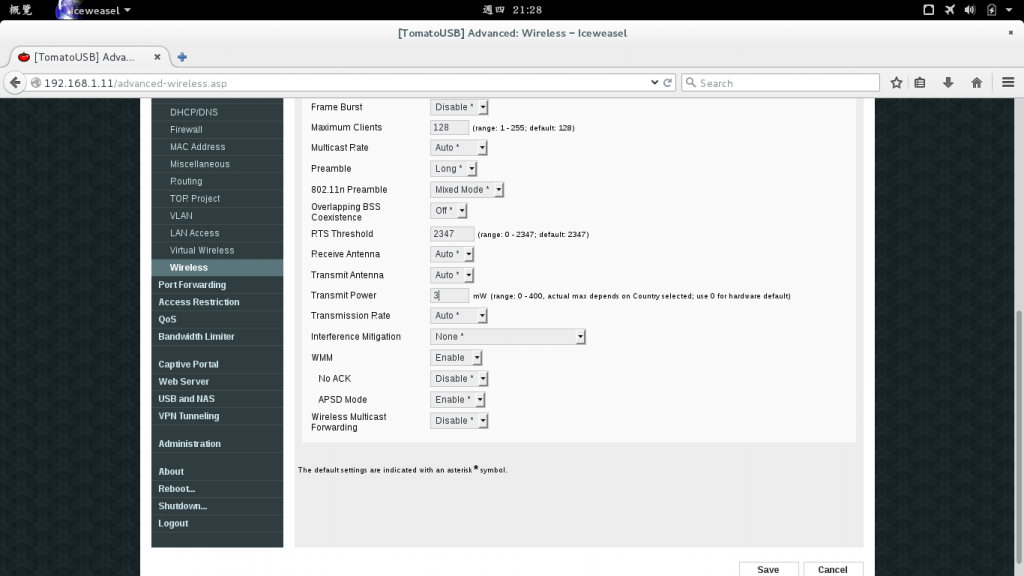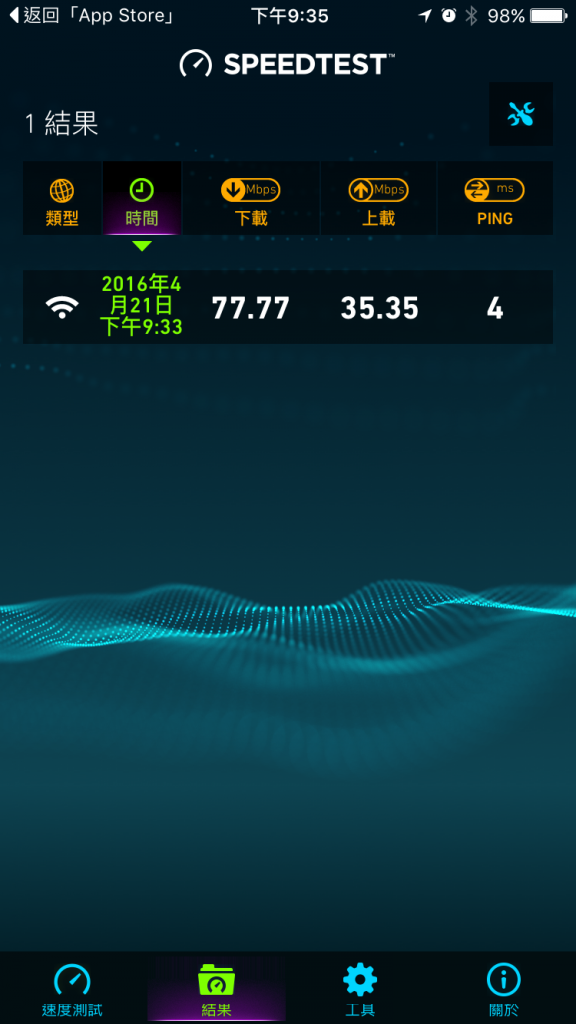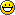# 無線網路功率3mW測速結果SpeedTest測速認證

P.S.

802.11n
11channel 2.462Ghz
20Mhz Width

AP Isolation: On can prevent WiFi local virus spreading
Beacon: 128ms
Distance/ACK: 24meter for home usage prevent UFO hack
DTIM Interval: 64ms
Fragmentation Threshold: 2304ms
RTS Threshold: 2295ms
Transmit Power: 3mW~5mW is enough for home usage
(Original Transmit Power Design is 42mW, Increase power when earth magnetic field in wrong direction. Must smaller than power design to avoid damage)

# 288*8=2304,288*255/256*8=2295
# This can load 288 solar energy in 256 phase/slice QAM
# 24meter is also related to solar wave packet!!
# 42mW in 2.4Ghz is also related to solar energy!!# 64ms, 128ms is related to solar frequency, when earth have a noise, it is also good!!

# Sad: Earth is not stable after moon or its spare come here.

GEM of the Earth
LCM(208,144)=1872
GCD(208,144)=16
Fragmentation Threshold=1872
RTS Threshold=1872-16=1856

GEM of the Solar
LCM(208,128)=1664
GCD(208,128)=16
Fragmentation Threshold=1664
RTS Threshold=1664-16=1648

GEM of the Galaxy to Sun
LCM(4096,208)=53248
GCD(4096,208)=16

GEM of the Galaxy to Earth
LCM(4096,288)=36864
GCD(4096,288)=32

Combine:
208 = 2^4 * 13
288 = 2^5 * 3^2
256 = 2^8
-> ( 2^8 * 3^2 * 13 = 29952 ) / ( 2^4 = 16 ) = 1876
Fragmentation Threshold=1876-sqrt(144)=1864
Fragmentation Threshold=1876
RTS Threshold=1876-19-13-11=1833
RTS Threshold=1876-13-11=1851
RTS Threshold=1876-11=1865
Orbit: Earth11, Venus13, Mercury19

Maximum Clients=128 *Maximum Concurrent Communication Object On The Track